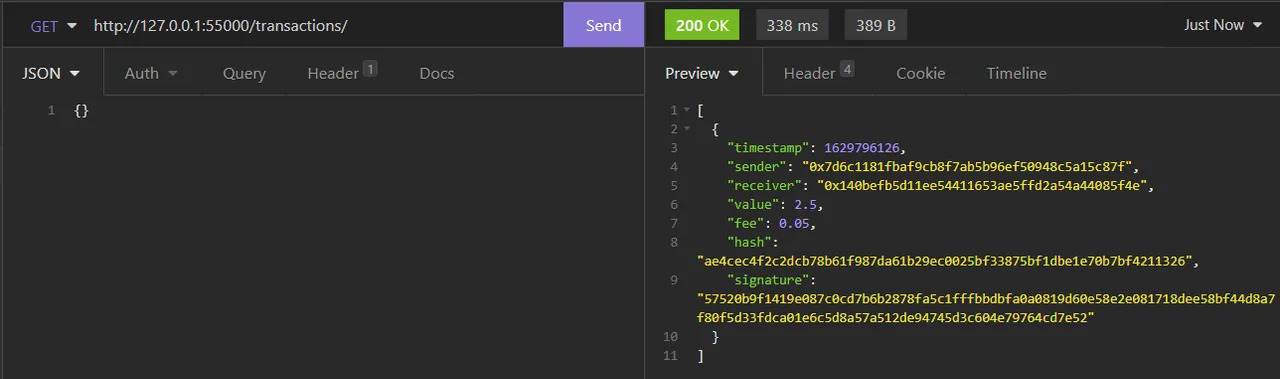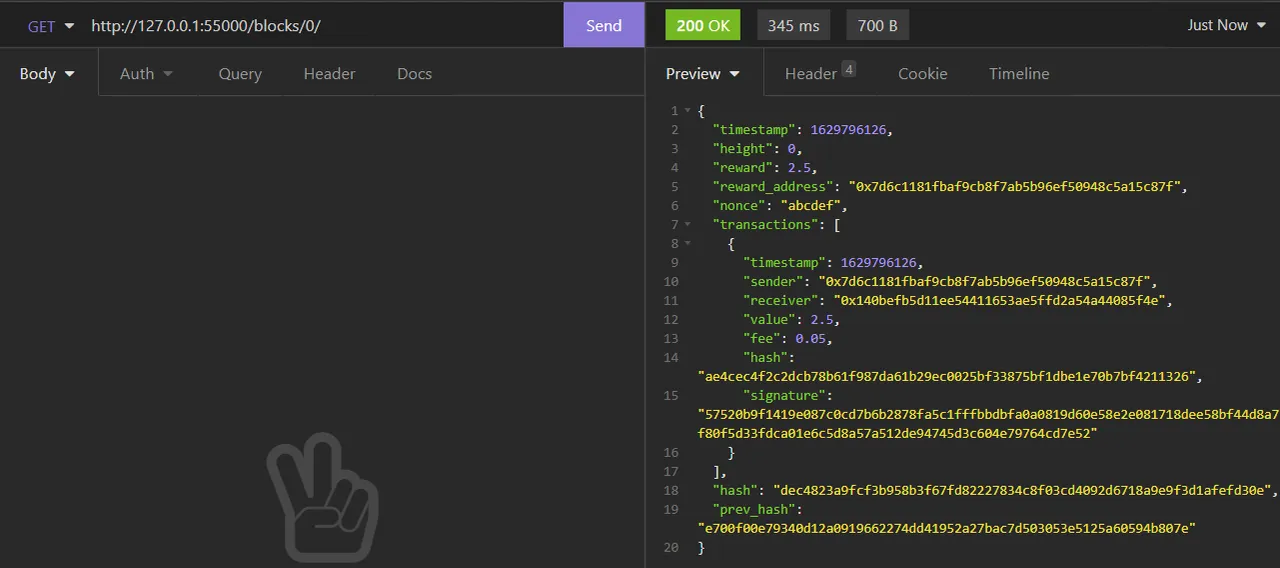# Programming - Implementing a Blockchain using Python and Flask [Dev Blog 5][Image 1]

## Introduction

Hey it's a me again drifter1!

Alrighty, I made two quite important additions this time around! The first one has to do with transaction hash calculation and signature verification. The second is about calculating and verifying the block hash.

In the case of transactions, the transaction.py script is extended with a function that allows for hash calculation. And of course, the transaction posting endpoint callback is extended to recalculate and check the hash, and verify the signature, before adding the transaction to the local file. Of course, balance checking is not yet implemented.

For the block structure, the block.py script's functionality is extended to be able to construct and destruct the block correctly to/from JSON format (yes, something was not working correctly there). For the block hash, simply turning the block data into a large byte-array (for now), hashing using SHA-256 is very easy, and so such a function is also included. The block creation endpoint callback now recalculates the hash and checks if it was calculated correctly, before creating the local block file. What is still necessary here is some form of consensus. Proof-of-work might be the best for getting started.

In order to check all this functionality easier a testing thread has been defined, which creates a correctly hashed and signed transaction, as well as a correctly hashed block. These are send to the corresponding local endpoints in order to check if they can truly be posted...

So, without further ado, let's get more in-depth!

## Transaction Hash Calculation

So, how does one go about calculating the hash for some structure? Well, in the case of JSON formatted data, we could simply turn the whole structure into the large JSON string and hash that! But, the field names (keys) are not important information, what's important is the actual transaction data. That's why its much better to turn only the information into bytes, or maybe even a large string. Using hexlify() and bytes() it's very easy to turn the data from the transaction into the bytes format as follows:

``````transaction_bytes = hexlify(bytes(str(transaction.timestamp), 'ascii'))
transaction_bytes += hexlify(bytes(transaction.sender, 'ascii'))
transaction_bytes += hexlify(bytes(str(transaction.value), 'ascii'))
transaction_bytes += hexlify(bytes(str(transaction.fee), 'ascii'))``````
and simply hashing these bytes using SHA-256 and taking the hex digest, gives us the hash:
``transaction.hash = sha256(transaction_bytes).hexdigest()``

## Transaction Verification

Up to this point, the callback of the transaction posting endpoint, simply checked for the correct JSON format. But, using the ECDSA functionality that was explained in the last Dev Blog, as well as the transaction hash calculation function, its now possible to check everything except the balance.

Recalculating and checking the hash is simply:

``````transaction = json_destruct_transaction(json_transaction)
calculate_transaction_hash(transaction)
if transaction.hash != json_transaction["hash"]:
# don't post transaction``````

Checking the signature can be done as follows:

``````signature = unhexlify(transaction.signature)
hash = unhexlify(transaction.hash)
if not verify_signature(signature, hash, transaction.sender):
# don't post transaction``````

Afterwards, we can again check if the transaction is not already included in the unconfirmed transactions (it might be the case if some delay occurred), and add the transaction to the local file. Here might also be a good point to send the transaction to all known nodes, but we will get into such tweaks later on, when the system is ready to roll...

## Block Hash Calculation and Verification

Let's skip the tweaks that I made in order to "print out" the block transactions correctly in JSON format...

The block hash can again be calculated using the same logic as with transactions. Use the hexlify() and bytes() functions in order to turn the block data into a large byte-array. In most implementations the transactions are not included one-by-one, but a total transactions hash is calculated before-hand, through merkle trees. But, let's for now simply put the transaction hash of each transaction into this large byte-array, as well.

The "draft" block hash calculation looks like this:

``````block_bytes = hexlify(bytes(str(block.timestamp), 'ascii'))
block_bytes += hexlify(bytes(str(block.height), 'ascii'))
block_bytes += hexlify(bytes(str(block.reward), 'ascii'))
block_bytes += hexlify(bytes(block.nonce, 'ascii'))
for transaction in block.transactions:
block_bytes += hexlify(bytes(transaction.hash, 'ascii'))
block_bytes += hexlify(bytes(block.prev_hash, 'ascii'))
block.hash = sha256(block_bytes).hexdigest()``````

So, in the block creation endpoint callback, recalculating and checking the hash is as simple as:

``````block = json_destruct_block(json_block)
calculate_block_hash(block)
if block.hash != json_block["hash"]:
# don't create block``````

This simply checks if the block is correctly hashed, it might also be wise to check each transaction again. And of course, a proof-of-work or proof-of-stake consensus is also necessary. We shall see, how things turn out...

Lastly, let's run the client.py script, so that I can explain what the testing thread does...

The testing thread code is as follows:

``````wallet_json = json.load(open(settings.wallet_path, "r"))
# create test transaction
transaction = Transaction(
value=2.5, fee=0.05)
calculate_transaction_hash(transaction)
private_key = json_retrieve_private_key(wallet_json)
sign_transaction(transaction, private_key)
local_post_transaction(settings, json_construct_transaction(transaction))
# create test block
nonce="abcdef", transactions=[transaction],
prev_hash="e700f00e79340d12a0919662274dd41952a27bac7d503053e5125a60594b807e")
calculate_block_hash(block)
json_block = json_construct_block(block)
local_create_block(settings, json_block)``````

You can see, that before the actual transaction is created, the wallet information is loaded from the local file. For the transaction, the sender is the wallet address, whilst the receiver is some random address. Using the transaction hash calculation function the corresponding hash is calculated and using the private key the transaction is signed. Afterwards, the transaction is sent to the local endpoint. Contacting the transaction retrieval endpoint using Insomnia, we should get back the corresponding transaction:If you try to send a badly hashed or signed transaction, the transaction will not be returned...

In the case of the block, the block is considered the first (height = 0), and the reward is defined to be sent to the wallet address. The nonce and previous hash are some random strings, and the only transaction is the transaction that was sent before. The block hash calculation function is used in order to calculate the block hash, and afterwards the block is sent to the local endpoint. Using Insomnia, retrieving the block yields:If the block hash is not correct, or some information is changed inside of the block, the block file is of course not created, and so no block would be retrieved...

## RESOURCES:

### Images

The rest is screenshots or made using draw.io

## Final words | Next up

And this is actually it for today's post!

I'm not quite sure if I will begin with balance-checking now (the UTXO-like structure) or get into the consensus first. We shall see...

I will keep you posted on my journey! Expect such articles to come out weekly, or even twice a week, until I'm finished!Keep on drifting!

H2
H3
H4
3 columns
2 columns
1 column